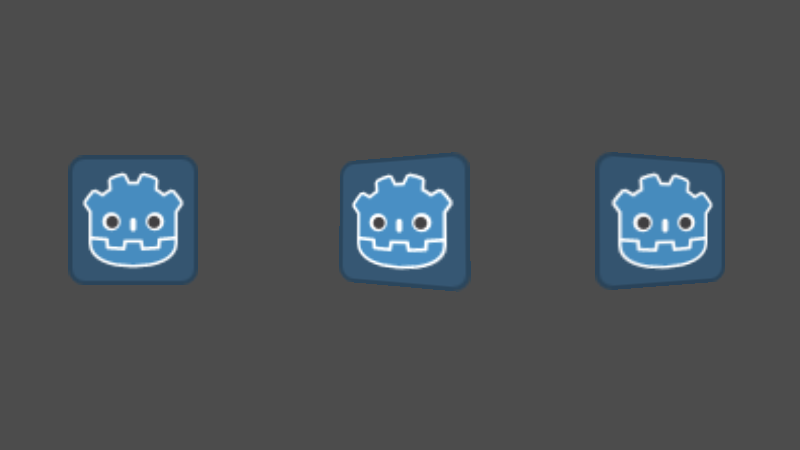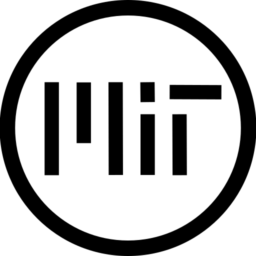# Sprite Perspective Correection

Add some depth with fake z-coordinate to 2d game.

Sample code:

``````extends Sprite

var mouse_on_card = false
var mouse_position_for_skew = Vector2(0, 0)

func _process(delta):
if not mouse_on_card:
# lerp position to (0, 0) if mouse outside bounds
mouse_position_for_skew = mouse_position_for_skew.linear_interpolate(Vector2(0, 0), 5 * delta )

func _input(event):
if event is InputEventMouseMotion:
var actual_rect = get_rect()
if actual_rect.has_point(to_local(event.position)):
mouse_on_card = true

mouse_position_for_skew = to_local(event.position)
else:
# if on previous motion mouse was on card and on this frame mouse is moved out - reset flag
if mouse_on_card:
mouse_on_card = false
``````

Put that code on sprite and add shader to sprite material. Points, closest to mouse position will appear deeper.

More sample code can be found here: https://github.com/veloc1/3dsprite

``````shader_type canvas_item;

uniform float width = 64;
uniform float height = 64;
uniform vec2 mouse_position = vec2(0, 0);

void fragment() {
vec2 uv = UV;

// map skew to [-0.5, 0.5]
float skew_x = mouse_position.x / width;
float skew_y = mouse_position.y / height;

// map to [-0.5, 0.5]
uv.x = (uv.x - 0.5);
uv.y = (uv.y - 0.5);

// ratio - how far are currnet point from mouse position
float sx = 1.0 - (uv.x * skew_x);
float sy = 1.0 - (uv.y * skew_y);

// calculate z (depth) depending on ratio
float z = 1.0 + (sx * sy) / 2.0;
// correct perspective for given point
uv.x = uv.x / z;
uv.y = uv.y / z;

// scale a bit down a reset mapping from [-0.5, 0.5] to [0, 1]
uv.x = uv.x / 0.45 + 0.5;
uv.y = uv.y / 0.45 + 0.5;
COLOR = texture(TEXTURE, uv);

// if uv outside texture - then use transparent color
if (uv.x < 0.0 || uv.x > 1.0) {
COLOR.a = 0.0;
} else if (uv.y < 0.0 || uv.y > 1.0) {
COLOR.a = 0.0;
} else {
// brightness
float brightness = 1.0 - mouse_position.y / (height / 2.0) * 0.2;
COLOR.rgb = texture(TEXTURE, uv, 1.0).rgb * brightness;

COLOR.a = texture(TEXTURE, uv, 1.0).a;
}
}``````
###### Tags
perspective, skew, spriteThe shader code and all code snippets in this post are under MIT license and can be used freely. Images and videos, and assets depicted in those, do not fall under this license. For more info, see our License terms.

### Perspective Grid Remix March2021Inline FeedbacksCoolmasterjake
1 year ago

Woah dude this shader is really cool! One thing that bothers me though is the complexity of the code, you can simple it down easily and get better results, so i thought i’d post it to help whoever needs it.

One thing that annoyed me with the sprite code is that when the mouse was on the left side of the sprite, the sprite looks down. Another thing you might want to do is change the brightness property in the shader and set 0.2 to something lower.

```extends Sprite

var lerpa :Vector2
var lerpi :Vector2
export var mouse_range = 120
export var speed_of_lerp = 0.1
var mouse

self.set_material(self.get_material().duplicate(true))

func _process(delta):
if get_global_mouse_position().distance_to(global_position) <mouse_range:
mouse = (get_global_mouse_position() - global_position)
lerpi = lerp(lerpi, mouse, speed_of_lerp)
if lerpi != mouse:
lerpa = lerpi
else:
lerpa = lerp(lerpa, Vector2.ZERO, speed_of_lerp)
if lerpa != Vector2.ZERO: